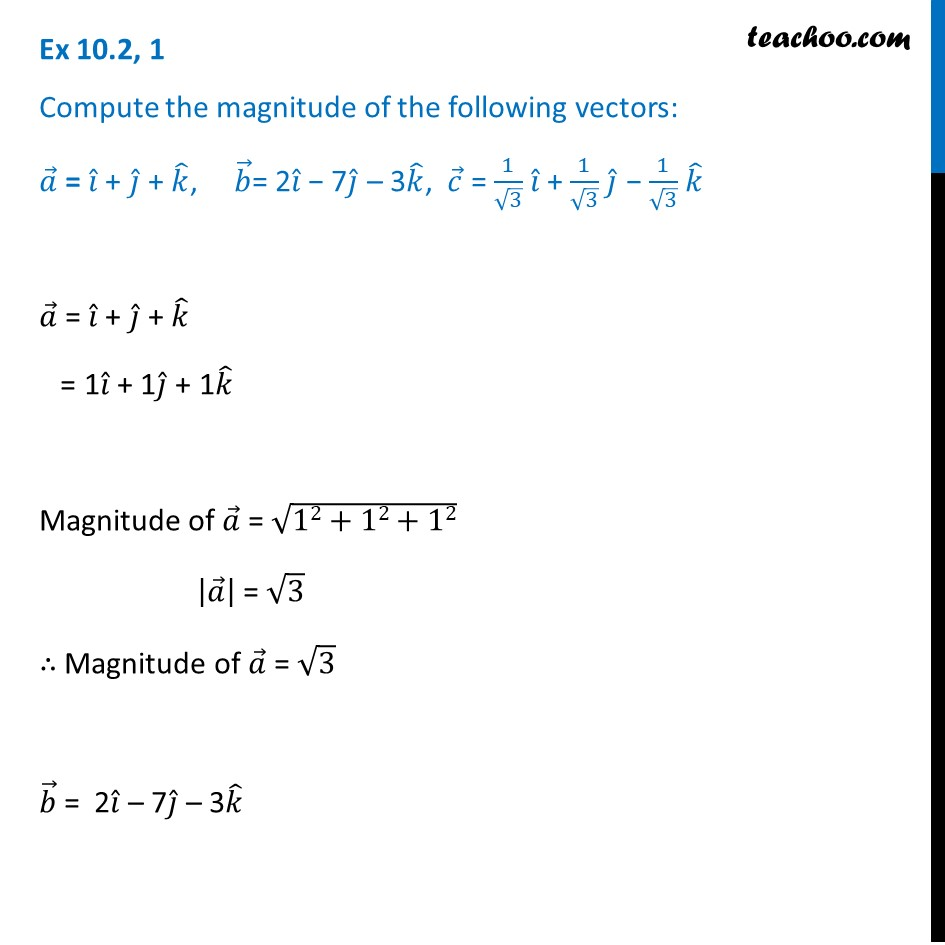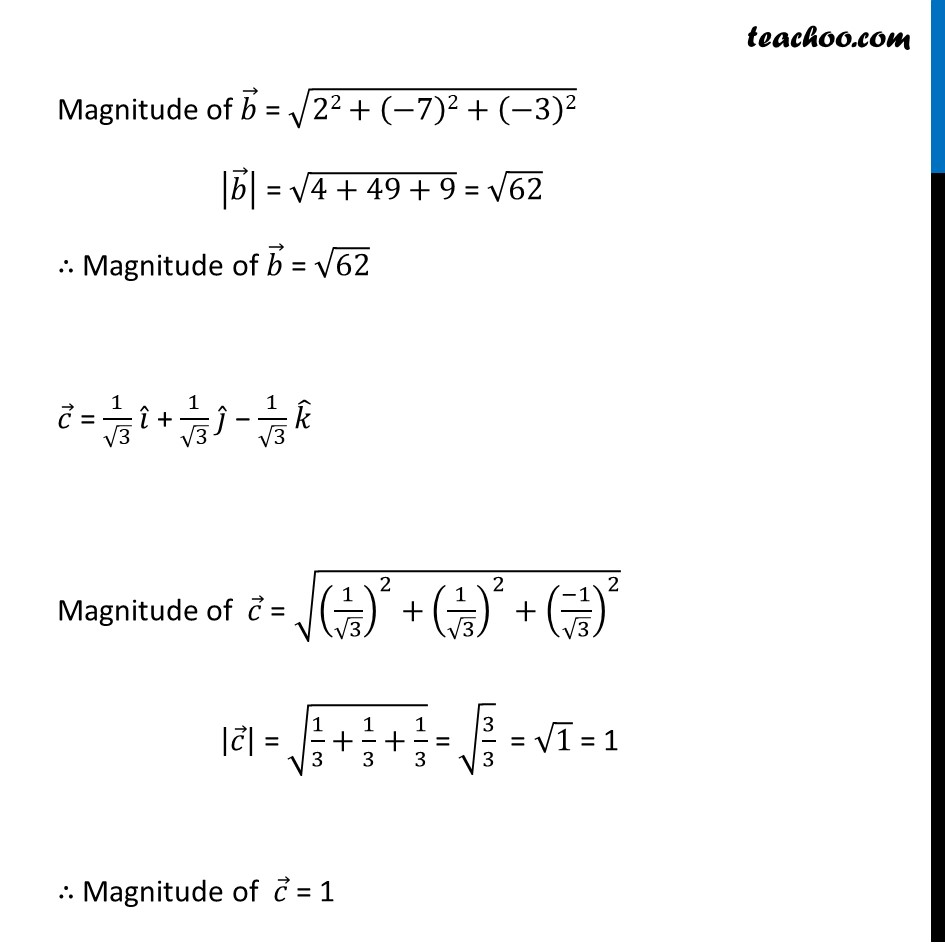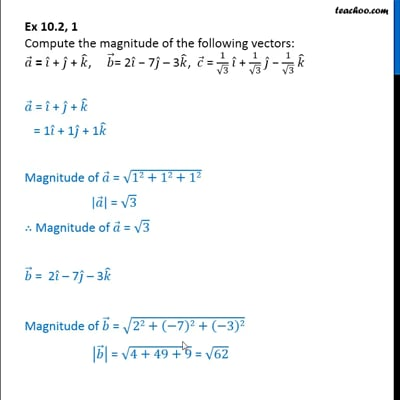Multiplication of a vector by a scalar

Chapter 10 Class 12 Vector Algebra
Concept wiseThis video is only available for Teachoo black users

Introducing your new favourite teacher - Teachoo Black, at only ₹83 per month

### Transcript

Ex 10.2, 1 Compute the magnitude of the following vectors: 𝑎 ⃗ = 𝑖 ̂ + 𝑗 ̂ + 𝑘 ̂, 𝑏 ⃗= 2𝑖 ̂ − 7𝑗 ̂ – 3𝑘 ̂, 𝑐 ⃗ = 1/√3 𝑖 ̂ + 1/√3 𝑗 ̂ − 1/√3 𝑘 ̂𝑎 ⃗ = 𝑖 ̂ + 𝑗 ̂ + 𝑘 ̂ = 1𝑖 ̂ + 1𝑗 ̂ + 1𝑘 ̂ Magnitude of 𝑎 ⃗ = √(12+12+12) |𝑎 ⃗ | = √3 ∴ Magnitude of 𝑎 ⃗ = √3 𝑏 ⃗ = 2𝑖 ̂ – 7𝑗 ̂ – 3𝑘 ̂ Magnitude of 𝑏 ⃗ = √(22+(−7)2+(−3)2) |𝑏 ⃗ | = √(4+49+9) = √62 ∴ Magnitude of 𝑏 ⃗ = √62 𝑐 ⃗ = 1/√3 𝑖 ̂ + 1/√3 𝑗 ̂ − 1/√3 𝑘 ̂ Magnitude of 𝑐 ⃗ = √((1/√3)^2+(1/√3)^2+((−1)/√3)^2 ) |𝑐 ⃗ | = √(1/3+1/3+1/3) = √(3/3) = √1 = 1 ∴ Magnitude of 𝑐 ⃗ = 1